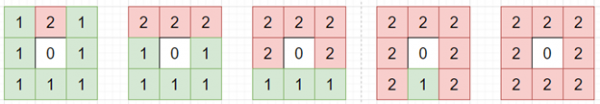# Program to find number of days it will take to burn all trees in python

Suppose we have a 2D matrix represents a forest where there are three types of cells: 0 empty cell 1 tree cell 2 tree on fire cell Every day, a tree catches fire when there is an adjacent (top, down, left, right, not diagonal) tree is on fire. We have to find the number of days it would take for every tree to be on fire. If that is not possible return -1.

So, if the input is like

 1 2 1 1 0 1 1 1 1

then the output will be 4,To solve this, we will follow these steps −

• ans := 0
• twos := a new list
• for i in range 0 to row count of matrix, do
• for j in range 0 to column count of matrix, do
• if matrix[i, j] is same as 2, then
• insert pair (i, j) at the end of twos
• while twos is not empty, do
• temp := a new list
• for each pair (i, j) in twos, do
• for each pair (x, y) in [(i + 1, j) ,(i, j + 1) ,(i - 1, j) ,(i, j - 1) ], do
• if x and y are in range of matrix and matrix[x, y] is 1, then
• insert pair (x, y) at the end of temp
• for each pair (i, j) in temp, do
• matrix[i, j] := 2
• twos := temp
• ans := ans + (1 if twos is not empty otherwise 0)
• ones = count number of 1s in matrix
• return ans if ones is 0 otherwise -1

Let us see the following implementation to get better understanding −

## Example

Live Demo

class Solution:
def solve(self, matrix):
ans = 0
twos = []
for i in range(len(matrix)):
for j in range(len(matrix)):
if matrix[i][j] == 2:
twos.append((i, j))
while twos:
temp = []
for i, j in twos:
for x, y in [(i + 1, j), (i, j + 1), (i - 1, j), (i, j - 1)]:
if 0 <= x < len(matrix) and 0 <= y < len(matrix) and matrix[x][y] == 1:
temp.append((x, y))
for i, j in temp:
matrix[i][j] = 2
twos = temp
ans += 1 if twos else 0

ones = sum(int(matrix[i][j] == 1) for i in range(len(matrix)) for j in range(len(matrix)))
return ans if ones == 0 else -1

ob = Solution()
matrix = [
[1, 2, 1],
[1, 0, 1],
[1, 1, 1]
]
print(ob.solve(matrix))

## Input

matrix = [
[1, 2, 1],
[1, 0, 1],
[1, 1, 1] ]

## Output

4